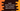# How to check if an array of integers contains two numbers appeared twice in swift## Introduction :

This post will show you how to check in an array of integers if it contains two numbers appeared twice or more than that using Swift. For example, if the array is [1,2,3,4,1,3,5], the program will print 1 and 3 as outputs.

## Solution :

The easiest way to solve this problem is by using a Dictionary. We will use the below algorithm to solve it :

1. Initialize one empty Int : Int dictionary i.e. the keys are integer and values are also integer in this dictionary.
2. Iterate over the array elements one by one.
3. For each number in the array, check if any key for that dictionary exists equal to the current number. If yes, increment its value by 1. Else, create one new key-value pairs with key equal to that number and value equal to zero.
4. Once the iteration will complete, we can check the dictionary to find out all the keys with value 2 or more than that.

## Swift program :

Let’s take a look at the program :

``````var m = [Int: Int]()

var arr: [Int] = [1,2,3,4,7,8,8,9,1,2]

for i in arr{
if let value = m[i] {
m[i] = value + 1
}else{
m[i] = 1
}
}

for i in m{
if(i.value > 1){
print(i.key)
}
}``````

It will print :

``````8
1
2``````